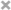# Kunpeng Song

Rutgers CS

Welcome to my blog~!

# Pytorch Summary sheet

## Tensor

### Tensor initialize

1. torch.empty(dim,dim); torch.zeros(dim,dim), torch.ones(dim,dim)
>>> torch.empty(5, 3)  # same as torch.zeros
tensor([[0., 0., 0.],
[0., 0., 0.],
[0., 0., 0.],
[0., 0., 0.],
[0., 0., 0.]])
>>> torch.ones(2,3)
tensor([[ 1.,  1.,  1.],
[ 1.,  1.,  1.]])

1. tensor.new_ones(dim,dim, dtype = tensor.dtype): Create a tensor based on an existing tensor. Reuse properties of the input tensor, e.g. dtype, unless new values are provided by user.
>>> x = torch.ones(2,3,dtype=torch.int8)  #default dtype is torch.float32
>>> x.dtype
torch.int8
>>> x.new_ones()
Traceback TypeError: ew_ones() missing 1 required positional arguments: "size" # need size
>>> x.new_ones(1, 3)
tensor([[ 1,  1,  1]], dtype=torch.int8)
>>> x.new_ones(1, 3, dtype=torch.double)
tensor([[ 1.,  1.,  1.]], dtype=torch.float64)

1. torch.tensor(list) ; torch.tensor(nparray)
2. torch.rand(dim,dim): random from a uniform distribution on the interval [0, 1) U(0,1)
>>> torch.rand(2, 3)
tensor([[0.5728, 0.5375, 0.0494],
[0.2820, 0.1853, 0.8619]]) #default dtype is torch.float32
>>> torch.rand(4)
tensor([ 0.5204,  0.2503,  0.3525,  0.5673])

1. torch.randn( size, dtype=None): random numbers from a normal distribution with mean 0 and variance 1 N(0,1)
>>> torch.randn(4)
tensor([-2.1436,  0.9966,  2.3426, -0.6366])
>>> torch.randn(2, 3)
tensor([[ 1.5954,  2.8929, -1.0923],
[ 1.1719, -0.4709, -0.1996]])
>>> torch.randn(2,2,3)
tensor([[[ 0.5827,  1.7046, -0.8720],
[ 0.2199,  0.5719,  1.9939]],
[[ 0.5509,  1.1897,  0.2434],
[-1.1203,  1.8258,  1.0824]]])
>>> torch.randn(2,1,3)
tensor([[[-0.2905,  0.0681, -0.8215]],
[[-0.4649,  0.5093,  0.5316]]])
>>> torch.randn(1,2,3)
tensor([[[-0.1372, -0.2624, -0.6892],
[-1.9984, -0.4962, -1.1629]]]) # pay attention to the shape of tensor

1. torch.randn_like(tensor, dtype = tensor.dtype); tensor.size(); tensor.shape

Returns a tensor with the same size as input that is filled with random numbers from a normal distribution N(0,1)

>>> x = torch.ones(2,3)
>>> x.dtype
torch.float32 #default dtype is torch.float32
>>> y = torch.randn_like(x) # do not need size
>>> y
tensor([[-0.0686,  1.9222, -1.7793],
[-2.1696,  1.5362,  0.0112]]) # same shape
>>> y.dtype
torch.float32 #same dtype as default
>>> z = torch.randn_like(x,dtype=torch.double) # specify double as dtype
>>> z
tensor([[ 1.0053,  0.8341,  2.8000],
[ 0.6961,  0.0417,  0.9913]], dtype=torch.float64) #double is torch.float64
>>> z.dtype
torch.float64
>>> z.size() # need (), not x.size!
torch.Size([1, 3]) # This is a tuple, so it supports all tuple operations.
>>> z.shape # do not need (). Same as numpy
torch.Size([1, 3]) # Same as size()

1. torch.manual_seed(num) :

>>> torch.manual_seed(2)
>>> print(torch.rand(2))#always same number. If not torch.manual_seed, different number

>>> Seed = random.randint(1, 10000)
>>> print(Seed)
6895
>>> print(random.seed(Seed))
>>> print(torch.manual_seed(Seed))
>>> print(random.random())
>>> print(torch.randn(1))
None
<torch._C.Generator object at 0x7ff6bed50d90>
0.14592352920524942
tensor([-1.0107])



# run these two rows in the same block, result is always same
>>> torch.manual_seed(Seed)
>>> torch.rand(2)
tensor([0.9825, 0.2720])

#run this line in the next jupyter notebook block, result keeps changing every time.
>>> torch.rand(2)
tensor([0.3530, 0.6419])

2. torch.arange(start=0, end, step=1) : same as tensor.range(), but it is deprecated (outdated).

>>> torch.arange(5)
tensor([ 0,  1,  2,  3,  4]) # torch.float32
>>> torch.arange(1, 4)
tensor([ 1,  2,  3])
>>> torch.arange(1, 2.5, 0.5)
tensor([ 1.0000,  1.5000,  2.0000])
>>> np.arange(5) # similar
array([0, 1, 2, 3, 4])
>>> torch.arange(5,11,2)
tensor([ 5.,  7.,  9.]) # notice: end excluded!

1. torch.randperm(n) : Returns a random permutation of integers from 0 to n - 1.
>>> torch.randperm(4)
tensor([2, 1, 0, 3])  # dtype = torch.int64

1. torch.tensor( list ): Construct a tensor directly from list
>>> torch.tensor([5.5, 3])
tensor([5.5000, 3.0000])

1. torch.cat ( ( tensor1, tensor2) ) # concat tensor, notice: tuple needed

>>> a = torch.tensor([1,2])
>>> b = torch.tensor([4,3])
>>> torch.cat((a,b,a))
tensor([1, 2, 4, 3, 1, 2])


more: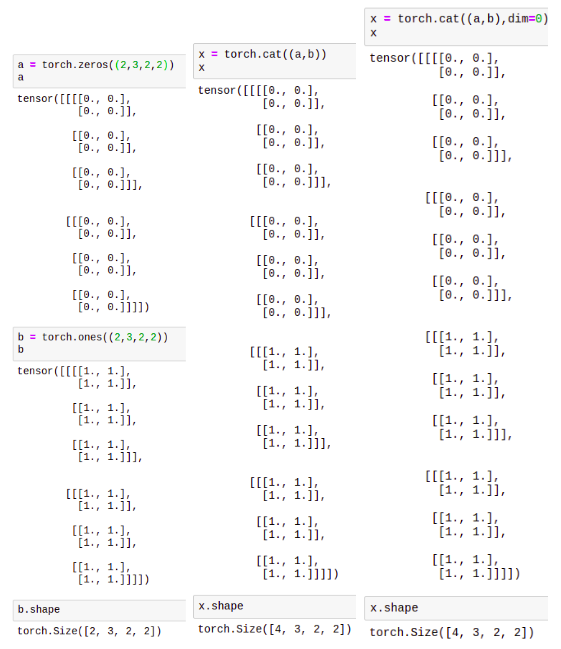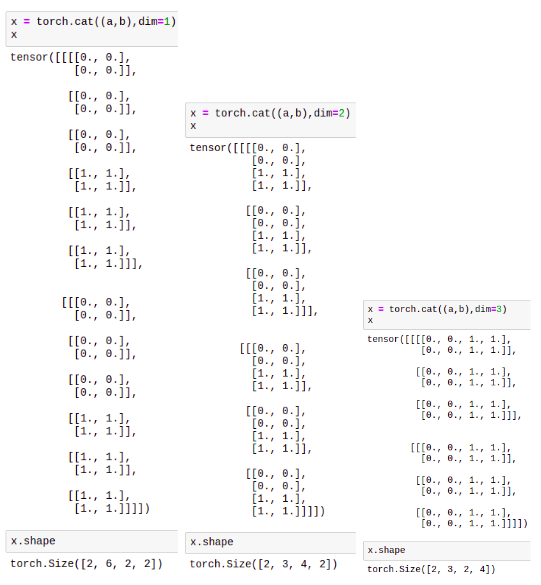2. torch.detach() or tensor1.detach()

Returns a new Tensor, detached from the current graph.

The result will never require gradient. Returned Tensor uses the same data tensor as the original one.

### Tensor iteration

1. torch.numel (tensor) : Returns the total number of elements in the input tensor. Same as a.numel()
>>> a = torch.randn(1, 2, 3, 4, 5) # same as  a.numel()
>>> torch.numel(a)
120
>>> a = torch.zeros(4,4)
>>> torch.numel(a)
16


### Tensor math

1.  + , and torch.add()
>>> x = torch.ones(2,3)
>>> y = torch.randn(2,3)
>>> x+y # same as torch.add(x,y)
tensor([[ 0.0066,  1.3231,  1.2001],
[ 1.1485, -0.0490,  2.0391]])
>>> z = torch.rand(2,3,dtype=torch.double) # different dtype. Same as torch.float64
>>> z+x
Traceback Expected object of type torch.DoubleTensor # cannot use +
# torch.add(x,z) also gives this Error


a = torch.randn(4)
>>> a
tensor([-0.9950,  1.6279, -1.5262, -0.1706])
tensor([ 19.0050,  21.6279,  18.4738,  19.8294]) # broadcast with scalar
>>> b = torch.randn(4)
>>> b
tensor([-0.8575, -0.2146,  0.6487,  0.6497])
tensor([-1.8525,  1.4133, -0.8775,  0.4791]) # same shape: broadcast with element
tensor([-9.5702, -0.5183,  4.9613,  6.3262]) # out = tensor1 + value × tensor2
>>> c = torch.randn(2,1)
>>> c
tensor([[ 1.7452],
[-2.0755]])
tensor([[ 0.7502,  3.3731,  0.2190,  1.5746], # different shape: broadcast to pair matix
[-3.0705, -0.4476, -3.6017, -2.2461]])


t1 = torch.randn(1, 3)
t2 = torch.randn(3, 1)
print(t1)
print(t2)
print('###')
print(t1+t2)
print('###')
t3 = torch.randn(3,3)
print(t3)
print(t1+t3)
>>>
tensor([[ 0.0989,  0.2018, -0.5701]])
tensor([[ 0.2082],
[-1.1686],
[ 0.0156]])
###
tensor([[ 0.3071,  0.4100, -0.3619], # broadcast to matrix
[-1.0696, -0.9667, -1.7387],
[ 0.1145,  0.2175, -0.5545]])
###
tensor([[-0.4906,  1.5755, -0.4574],
[-2.2614, -0.9916, -1.6035],
[-0.2944, -1.4336,  0.1889]])
tensor([[-0.3917,  1.7774, -1.0275], # 1*3 nultiple with 3*3: broadcast to 3*3
[-2.1625, -0.7898, -2.1736],
[-0.1954, -1.2318, -0.3813]])


Some variations:

• torch.addcmul(tensor, value=1, tensor1, tensor2) : outi = tensori + value × tensor1i × tensor2i
• torch.addcdiv(tensor, value=1, tensor1, tensor2) : outi = tensori + value × tensor1i / tensor2i

Other element wise math function

1. torch.abs(tensor) : element-wise absolute value of the given input tensor. Same as tensor.abs()

2. sin cos tan asin acos atan

3. torch.ceil(tensor) : Returns a new tensor with the ceil of the elements of input, the smallest integer greater than or equal to each element.

4. torch.floor(tensor) : similar as before but smaller than original value; torch.round(tensor) : closet integer

5. torch.clamp(tensor, min = min, max = max) :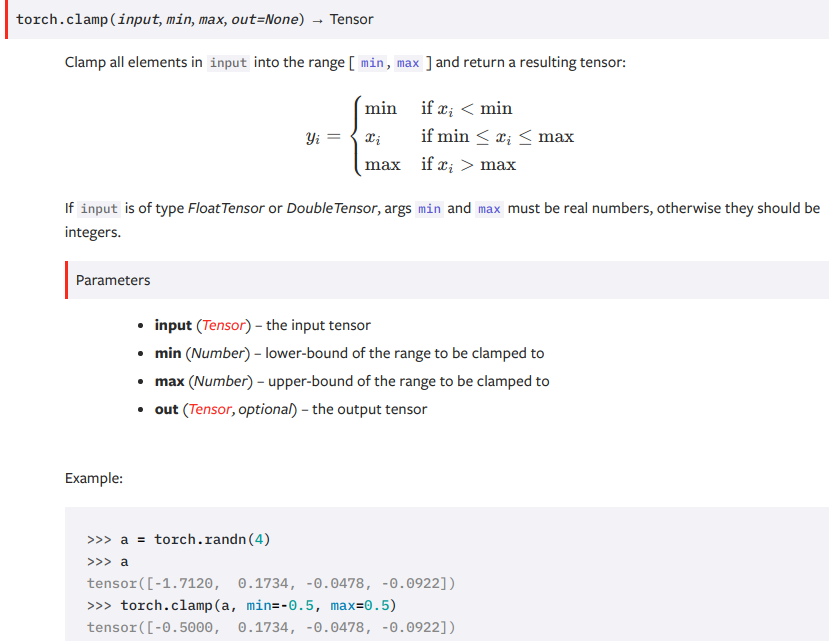6. torch.clamp(tensor, min = min) : Clamps all elements in input to be larger or equal min. (Similarly, max)

7. torch.mul(tensor, value) ; torch.mul(tensor1, tensor2); Similarly div

8. torch.exp(tensor) : e^tensori

9. torch.fmod(tensor, value) : Computes the element-wise remainder of division.

10. torch.frac(tensor) : Computes the fractional portion of each element in input.

torch.frac(torch.tensor([1, 2.5, -3.2]))
tensor([ 0.0000,  0.5000, -0.2000])

11. torch.lerp(start, end, weigth) : Does a linear interpolation of two tensors start and end based on a scalar weight and returns the resulting outtensor. outi=starti+weight×(endi−starti)

>>> start = torch.arange(1., 5.)
>>> end = torch.empty(4).fill_(10)
>>> start
tensor([ 1.,  2.,  3.,  4.])
>>> end
tensor([ 10.,  10.,  10.,  10.])
>>> torch.lerp(start, end, 0.5)
tensor([ 5.5000,  6.0000,  6.5000,  7.0000])

12. torch.log : log e ; torch.log10 : log 10 ; torch.log2 : log 2 ; torch.neg : negative

13. torch.power(tensor, value) : power by value ; torch.power(tensor, tensor) : power by pair tensor

14. torch.reciprocal(tensor) : 1 / tensor

15. torch.reminder(tensor, divisor) : Computes the element-wise remainder of division. (divisor: value or tensor)

16. torch.sqrt(tensor) : sqrt(tensor) ; torch.rsqrt(tensor) : 1 / sqrt(tensor)

17. torch.sigmoid(tensor) :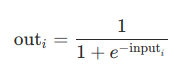18. torch.sign(tensor) : 1, -1, 0

19. torch.trunc(tensor) : Returns a new tensor with the truncated integer values of the elements of input.

>>> a = torch.randn(4)
>>> a
tensor([ 3.4742,  0.5466, -0.8008, -0.9079])
>>> torch.trunc(a)
tensor([ 3.,  0., -0., -0.]) # simply delet fraction


### Reductions

1. torch.argmax(tensor, dim=None) : Returns the indices of the maximum values of a tensor across a dimension.

>>> a = torch.randn(3, 4)
>>> a
tensor([[ 0.7913,  0.9236,  0.0348,  0.5403],
[ 0.4394, -0.4464, -0.6759, -0.3718],
[-1.4133,  1.0612,  1.0322, -2.5048]])
>>> torch.argmax(a) # argmax in the flatten tensor
tensor(9)
>>> torch.argmax(a, dim=0) # col
tensor([ 0,  2,  2,  0])
>>> torch.argmax(a, dim=1) # row
tensor([ 1,  0,  1])
# same as argmin

2. Torch.prod(tensor,dim=None) : product of all elements

3. torch.cumprod(tensor,dim,dtype=None) : cumulative product of elements of input in the dimension dim

>>> a = torch.randn(7)
>>> a
tensor([ 0.6001,  0.2069, -0.1919,  0.9792,  0.6727,  1.0062,  0.4126])
>>> torch.cumprod(a, dim=0)
tensor([ 0.6001,  0.1241, -0.0238, -0.0233, -0.0157, -0.0158, -0.0065])
>>> a = 0.0
>>> torch.cumprod(a, dim=0) # cumulative. So, from 5th on, there are all zeros
tensor([ 0.6001,  0.1241, -0.0238, -0.0233, -0.0157, -0.0000, -0.0000])

>>> a = torch.randn(3,4)
>>> a[0,2] = 0
>>> a[2,1] = 0
>>> a
tensor([[-0.4318,  0.4427,  0.0000, -0.1199],
[-0.2790,  0.9716, -0.9334,  1.1384],
[-1.1763,  0.0000, -1.4534, -1.2983]])
>>> torch.cumprod(a,dim=0) # col
tensor([[-0.4318,  0.4427,  0.0000, -0.1199],
[ 0.1205,  0.4302, -0.0000, -0.1365],
[-0.1417,  0.0000,  0.0000,  0.1773]])
>>> torch.cumprod(a,dim=1) # row
tensor([[-0.4318, -0.1912, -0.0000,  0.0000],
[-0.2790, -0.2711,  0.2530,  0.2880],
[-1.1763, -0.0000,  0.0000, -0.0000]])

4. torch.cumsum(tensor, dim, dtype = None) : similar as cumprod

5. torch.dist(tensor1, tensor2, p=2) : Returns the p-norm (Frobenius norm) of (input - other), it is a scalar value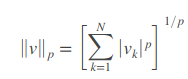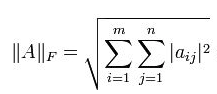6. torch.mean(tensor, dim=None, keepdim =False) :

>>> a = torch.rand(3,2)
>>> a
tensor([[ 0.5877,  0.6770],
[ 0.9455,  0.4647],
[ 0.0451,  0.9079]])
>>> torch.mean(a)
tensor(0.6047)
>>> torch.mean(a,dim=1)
tensor([ 0.6323,  0.7051,  0.4765])
>>> torch.mean(a,1) #same above
tensor([ 0.6323,  0.7051,  0.4765])
>>> torch.mean(a, 1, True) #keep dimension
tensor([[ 0.6323],
[ 0.7051],
[ 0.4765]])
>>> torch.mean(a,dim=0)
tensor([ 0.5261,  0.6832])
# similarly: torch.median();

7. torch.norm(tensor, p=’fro’, dim=None, keepdim=False, out=None) : Returns the matrix norm8. torch.std(tensor, dim=None) : Returns the standard-deviation of all elements in the input tensor. Similarly: var()

9. torch.sum(tensor, dim, keepdim=False) : Returns the sum of each row of the input tensor in the given dimension dim. If dim is a list of dimensions, reduce over all of them. (dim = tuple is disabled on iLab)

10. torch.unique(tensor,sorted=False,return_inverse=False,dim=None) : Returns the unique scalar elements of the input tensor as a 1-D tensor.

• sorted (bool) – Whether to sort the unique elements in ascending order
• return_inverse (bool) – Whether to also return the indices for where elements in the original input ended up in the returned unique list.
>>> output = torch.unique(torch.tensor([1, 3, 2, 3], dtype=torch.long))
>>> output
tensor([ 2,  3,  1])
>>> a = torch.tensor([[1, 3], [2, 3]]
>>> output, inverse_indices = torch.unique(a, sorted=True, return_inverse=True)
>>> output
tensor([ 1,  2,  3])
>>> inverse_indices
tensor([[ 0,  2],
[ 1,  2]])

11. A LOT OF FUNCTIONS. SUMMARIZE LATER.

12. torch.matmul(tensor1,tensor2) : Matrix multiplication of two tensors. Cases when they have different dimensions:

• If tensor1 is 1-D, tensor2 is 2_D: 1 is prepended to its dimension for the purpose of the matrix multiply (Turn a vector to matrix). After the matrix multiply, the prepended dimension is removed (turn back to vector).
• If tensor1 is 2-D, tensor2 is 1_D: matrix-vector product
• At least one is N-D: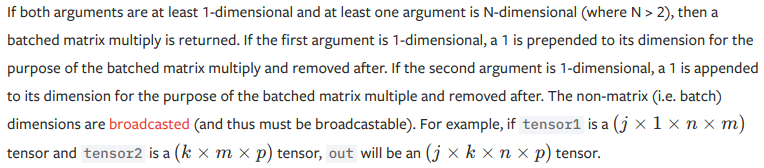>>> a = torch.rand(3)
>>> b = torch.rand(3)
>>> torch.matmul(a,b) # vector * vector
tensor(0.2872) # size() = torch.Size([])

>>> a = torch.rand(3,4)
>>> b = torch.rand(4)
>>> torch.matmul(a,b).size() # matrix * vector
torch.Size()

>>> a = torch.rand(3)
>>> b = torch.rand(3,4)
>>> torch.matmul(a,b) # vector * matrix
tensor([ 1.1128,  0.9645,  0.6328,  1.0789])
# TO show the above more clearly:
>>> torch.tensor([[1,2,3]]).size() # This is matrix
torch.Size([1, 3])
>>> torch.tensor([1,2,3]).size() # This is vector
torch.Size()
# And vector is different from matrix:
# (1)
>>> a = tensor([ 1,  2,  3])
>>> b = tensor([[ 1,  2], [ 3,  4],[ 4,  5]])
>>> torch.matmul(a,b)
tensor([[ 19,  25]])
# (2)
>>> a = tensor([[ 1,  2,  3]])
>>> b = tensor([[ 1,  2], [ 3,  4],  [ 4,  5]])
>>> torch.matmul(a,b)
tensor([ 19,  25])

>>> a = torch.rand(3,4)
>>> b = torch.rand(4,5)
>>> torch.matmul(a,b).size()
torch.Size([3, 5])

>>> # batched matrix x broadcasted vector
>>> tensor1 = torch.randn(10, 3, 4)
>>> tensor2 = torch.randn(4)
>>> torch.matmul(tensor1, tensor2).size()
torch.Size([10, 3])
>>> # batched matrix x batched matrix
>>> tensor1 = torch.randn(10, 3, 4)
>>> tensor2 = torch.randn(10, 4, 5)
>>> torch.matmul(tensor1, tensor2).size()
torch.Size([10, 3, 5])
>>> # batched matrix x broadcasted matrix
>>> tensor1 = torch.randn(10, 3, 4)
>>> tensor2 = torch.randn(4, 5)
>>> torch.matmul(tensor1, tensor2).size()
torch.Size([10, 3, 5])

# To understand the dimensionality of tensor:
#	a torch.Size([10, 3, 4]) : 10 layer, 3 rows per layer, 4 elements per row
#	b torch.Size([10, 4, 5]) : 10 layer, 4 rows per layer, 5 elements per row
#	torch.matmul(a, b) : layer by layer matrix mul. --> torch.Size([10, 3, 5])
#	c torch.Size([4,5]) : 2-D --> 1 layer 3-D.
#	torch.matmul(a, c) : c boradcast. layer by layer mul. torch.Size([10, 3, 5])

13. torch.mm(mat1, mat2) : matrix multiplication of the matrices mat1 and mat2. (m x n) (n x p) –> (m x p)14. torch.mv(mat, vec) : matrix-vector product. mat is (n×m), vec1-D of size m –> 1-D of size n. Not broadcastable.

15. torch.addmm(beta=1,mat,alpha=1,mat1,mat2) : matrix multiplication (Not element wise) of the matrices mat1 and mat2. The matrix mat is added to the final result.

• out=β mat+α (mat1i * mat2i)
• alpha, beta are optional. But mat is required.
>>> a = torch.rand(2,4)
>>> b = torch.rand(4,3)

16. torch.addmv(beta=1, tensor, alpha=1, mat, vec) : matrix-vector product of the matrix mat and the vector vec. The tensor is added to the final result.

• out=β tensor+α (mat * vec)
• alpha, beta are optional. But tensor is required.
17. torch.addr(beta=1, mat, alpha=1, vec1, vec2) : outer-product of vectors vec1 and vec2 and adds it to matrix mat

• out=β mat+α (vec1⊗vec2)
• If vec1 is a vector of size n and vec2 is a vector of size m, then mat must be broadcastable with a matrix of size (n x m) and out will be a matrix of size(n×m).
>>> a = torch.arange(1,4) # a = tensor([ 1.,  2.,  3.]) it is 1-D
>>> b = torch.arange(1,3)
tensor([[ 1.,  2.],
[ 2.,  4.],
[ 3.,  6.]])
##attention: The following won't work!
>>> a = torch.rand(1,4) # This is a 2_D matrix. It is not a 'vector'
>>> b = torch.rand(1,3)
Traceback Expected 1-D argument vec1, but got 2-D

18. torch.dot(tensor1,tensor2) : dot product (inner product) of two tensors. Returning is a scalar tensor.

19. torch.squeeze(input, dim=None, out=None) : Returns a tensor with all the dimensions of input of size 1 removed.

• If input is of shape: (A \times 1 \times B \times C \times 1 \times D)(A×1×B×C×1×D) then the out tensor will be of shape: (A \times B \times C \times D)(A×B×C×D).
• When dim is given, a squeeze operation is done only in the given dimension. If input is of shape: (A \times 1 \times B)(A×1×B),squeeze(input, 0) leaves the tensor unchanged, but squeeze(input, 1) will squeeze the tensor to the shape (A \times B)(A×B).
>>> a = torch.randn(1,2,2,2)
>>> a
tensor([[[[-0.3458,  1.3588],
[-0.2800, -1.1741]],
[[-0.9958, -1.0715],
[-0.0628, -0.3520]]]])
>>> a.view(-1, 1)
tensor([[-0.3458],
[ 1.3588],
[-0.2800],
[-1.1741],
[-0.9958],
[-1.0715],
[-0.0628],
[-0.3520]])
>>> a.view(-1, 1).shape
torch.Size([8, 1])
>>> a.view(-1, 1).squeeze(1)
# tensor.squeeze(dim=None)  SAME AS torch.squeeze(tensor,dim=None)
tensor([-0.3458,  1.3588, -0.2800, -1.1741, -0.9958, -1.0715, -0.0628,
-0.3520])


more: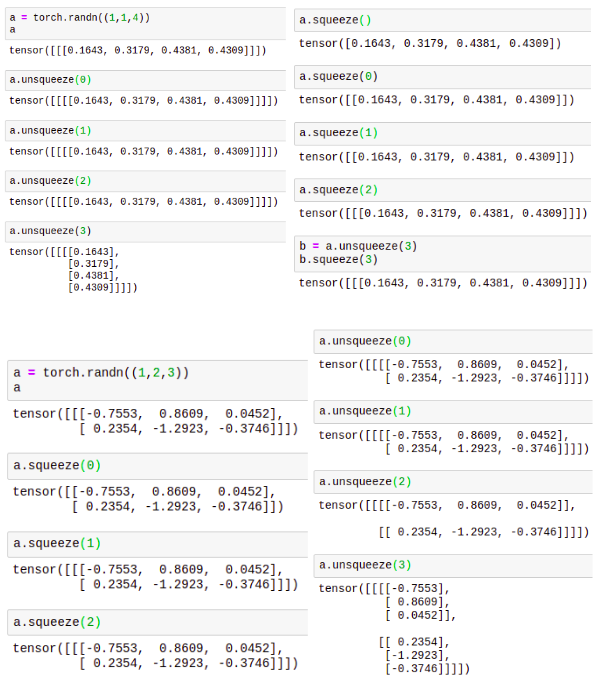20. torch.tensor.item() : Returns the value of this tensor as a standard Python number. This only works for tensors with one element.

>>> x = torch.tensor([1.0]) # only for tensors with one element.
>>> x.item()
1.0

21. torch.tensor.tolist() : Returns the tensor as a (nested) list. Tensors are automatically moved to the CPU first.

22. tensor1.expand( tensor.size() ) :

Returns a new view of the self tensor with singleton dimensions expanded to a larger size, without allocating new memory. Passing -1 as the size for a dimension means not changing the size of that dimension. Tensor can be also expanded to a larger number of dimensions, and the new ones will be appended at the front. For the new dimensions, the size cannot be set to -1.

>>> x = torch.Tensor([, , ])
>>> x.size()
torch.Size([3, 1])
>>> x.expand(3, 4)
1  1  1  1
2  2  2  2
3  3  3  3
[torch.FloatTensor of size 3x4]
>>> x.expand(-1, 4)   # -1 means not changing the size of that dimension
1  1  1  1
2  2  2  2
3  3  3  3
[torch.FloatTensor of size 3x4]
>>> x.expand(2,3,4) # add new dim at the very outside dim
tensor([[[1., 1., 1., 1.],
[2., 2., 2., 2.],
[3., 3., 3., 3.]],

[[1., 1., 1., 1.],
[2., 2., 2., 2.],
[3., 3., 3., 3.]]])
>>> y = torch.tensor([1,2,3,4])
>>> y
tensor([1, 2, 3, 4])
>>> y.expand(3,3,-1)
tensor([[[1, 2, 3, 4],
[1, 2, 3, 4],
[1, 2, 3, 4]],

[[1, 2, 3, 4],
[1, 2, 3, 4],
[1, 2, 3, 4]],

[[1, 2, 3, 4],
[1, 2, 3, 4],
[1, 2, 3, 4]]])

23. torch.transpose( tensor, dim0, dim1) :

Returns a tensor that is a transposed version of input. The given dimensions dim0 and dim1 are swapped.

>>> x = torch.randn(2, 3)
>>> x
tensor([[ 1.0028, -0.9893,  0.5809],
[-0.1669,  0.7299,  0.4942]])
>>> torch.transpose(x, 0, 1)
tensor([[ 1.0028, -0.1669],
[-0.9893,  0.7299],
[ 0.5809,  0.4942]])


Notice: the returned tensor is not contiguous and cannot be applied torch.view()

24. tensor.contiguous() :

Some tensor is constructed by several tensor blocks, rather than an contiguous memory block. These tensors can not be applied torch.view(). And need to be transferred to contiguous tensor beforehand.

>>> x = torch.Tensor([, , ])
tensor([[1.],
[2.],
[3.]])
>>> y = x.expand(2,3,4)
>>> y
tensor([[[1., 1., 1., 1.],
[2., 2., 2., 2.],
[3., 3., 3., 3.]],

[[1., 1., 1., 1.],
[2., 2., 2., 2.],
[3., 3., 3., 3.]]])
>>> y.is_contiguous()
False
>>> y.view(3,-1)
RuntimeError: invalid argument 2: view size is not compatible with input tensor size and stride (at least one dimension spans across two contiguous subspaces)
>>> y.contiguous().view(3,-1)
tensor([[1., 1., 1., 1., 2., 2., 2., 2.],
[3., 3., 3., 3., 1., 1., 1., 1.],
[2., 2., 2., 2., 3., 3., 3., 3.]])

25. torch.numel( tensor ) : equals : torch.nelement( tensor )

Returns the total number of elements in the input tensor.

>>> a = torch.randn(1, 2, 3, 4, 5)
>>> torch.numel(a)
120

26. To be continued.

## NN

To be continued.

References:

 Pytorch: tensor https://pytorch.org/docs/stable/torch.html#torch.fmod

1. torch.backends.cudnn.benchmark = True #enables benchmark mode in cudnn.inbuilt cudnn auto-tuner to find the best algorithm to use for your hardware.

2. import argparse
parser = argparse.ArgumentParser()
parser.add_argument('--dataset', required=True, help='cifar10 |lsun |mnist |imagenet')
parser.add_argument('--batchSize', type=int, default=64, help='input batch size')
opt = parser.parse_args()

root = opt.dataroot
...

3. dataset = torchvision.datasets.ImageFolder('path/to/imagenet_root/')
'''
'''
batch_size=4,
shuffle=True,


Use some common datasets and generate a data loader (https://pytorch.org/docs/stable/torchvision/datasets.html#imagefolder)

4. torch.utils.data.DataLoader(dataset, batch_size=1, shuffle=False, sampler=None, batch_sampler=None, num_workers=0) : Retrun (batch_x, batch_y)

import torch.utils.data as Data
torch.manual_seed(1)    # reproducible
BATCH_SIZE = 5
x = torch.linspace(1, 10, 10)       # x data (torch tensor)
y = torch.linspace(10, 1, 10)       # y data (torch tensor)
# conver to torch Dataset
torch_dataset = Data.TensorDataset(data_tensor=x, target_tensor=y)
dataset=torch_dataset,      # torch TensorDataset format
batch_size=BATCH_SIZE,      # mini batch size
shuffle=True,
num_workers=2,
)
for epoch in range(3):   # all training data 3 iterations
for step, (batch_x, batch_y) in enumerate(loader):  #
# TRAINING CODE
print('Epoch: ', epoch, '| Step: ', step, '| batch x: ',
batch_x.numpy(), '| batch y: ', batch_y.numpy())
"""
Epoch:  0 |Step:  0 |batch x:[ 6.  7.  2.  3.  1.] | batch y:[  5.   4.   9.   8.  10.]
Epoch:  0 |Step:  1 | batch x:[  9.  10.   4.   8.  5.] | batch y:[ 2.  1.  7.  3.  6.]
Epoch:  1 |Step:  0 | batch x:  [  3.  4.  2.  9.  10.] | batch y:[ 8.  7.  9.  2.  1.]
Epoch:  1 |Step:  1 | batch x:  [ 1.  7.  8.  5.  6.] | batch y [ 10.  4.  3.  6.   5.]
Epoch:  2 |Step:  0 | batch x:  [ 3.  9.  2.  6.  7.] | batch y:  [ 8.  2.  9.  5.  4.]
Epoch:  2 |Step:  1 | batch x:  [ 10.  4.  8.  1.  5.] | batch y:[  1.  7.  3. 10.  6.]
"""


https://morvanzhou.github.io/tutorials/machine-learning/torch/3-05-train-on-batch/

5. >>> w = torch.empty(3, 5)
>>> nn.init.normal_(w)


Fills the input Tensor with values drawn from the normal distribution

6. x = torch.randn(3, requires_grad=True)

>>> out
True
True
False # The wrapper "with torch.no_grad()" temporarily set all the requires_grad flag to false.

7. weight initialize : define your name check function, which applies selectively the initialisation.

def weights_init(m):
classname = m.__class__.__name__
if classname.find('Conv') != -1:
xavier(m.weight.data)
xavier(m.bias.data)
# Then traverse the whole set of Modules.
net = Net() # generate an instance network from the Net class
net.apply(weights_init) # apply weight init

# another eg:
# custom weights initialization called on netG and netD
def weights_init(m):
classname = m.__class__.__name__
if classname.find('Conv') != -1: # if is conv: normalize to N(0,0.02)
m.weight.data.normal_(0.0, 0.02)
elif classname.find('BatchNorm') != -1: # if is BN: normalize to N(1,0.02)
m.weight.data.normal_(1.0, 0.02)
m.bias.data.fill_(0) # also init bias
netG = Generator(ngpu).to(device)
netG.apply(weights_init)
netD = Discriminator(ngpu).to(device)
netD.apply(weights_init)

# others:
#check if some module is an instance of a class
def weights_init(m):
if isinstance(m, nn.Conv2d):
xavier(m.weight.data)
xavier(m.bias.data)

8. nn.relu(inplace=False) :

inplace – can optionally do the operation in-place, modifying the input directly, without allocating any additional output. It can sometimes slightly decrease the memory usage, but may not always be a valid operation. Default: False

>>> m = nn.ReLU()
>>> tensor = torch.randn(2)
>>> output = m(tensor)

9. nn.Conv2d(in_channels, out_channels, kernel_size, stride=1, padding=0, dilation=1, groups=1, bias=True) :

>>> a = torch.randn(1,3,3,3)
>>> conv1 = torch.nn.Conv2d(3,1,3,1,0) # no padding
>>> conv1(a).size()
torch.Size([1, 1, 1, 1])
>>> conv2 = torch.nn.Conv2d(3,1,3,1,1) # padding by FLOOR(kernel_size/2)
>>> conv2(a).shape
torch.Size([1, 1, 3, 3])
>>> conv3 = torch.nn.Conv2d(3,1,3,1) # default is no-padding
>>> conv3(a).shape
torch.Size([1, 1, 1, 1])

10. nn.ConvTranspose2d (in_channels, out_channels, kernel_size, stride=1, padding=0, output_padding=0, groups=1, bias=True, dilation=1) :

This module can be seen as the gradient of Conv2d with respect to its input. It is also known as a fractionally-strided convolution or a deconvolution (although it is not an actual deconvolution operation).

>>> a = torch.randn(1, 16, 12, 12)

>>> downsample = nn.Conv2d(16, 16, 3, stride=2)
>>> b = downsample(a)
>>> b.size()
torch.Size([1, 16, 5, 5])

>>> downsample = nn.Conv2d(16, 16, 3, stride=2,padding=1)
>>> b = downsample(a)
>>> b.size()
torch.Size([1, 16, 6, 6])

>>> upsample = nn.ConvTranspose2d(16, 16, 3, stride=2)
>>> b = upsample(a)#, output_size=input.size())
>>> b.size()
torch.Size([1, 16, 25, 25])

>>> upsample = nn.ConvTranspose2d(16, 16, 3, stride=2, padding=1)
>>> b = upsample(a)#, output_size=input.size())
>>> b.size()
torch.Size([1, 16, 23, 23])

11. conv_kernel size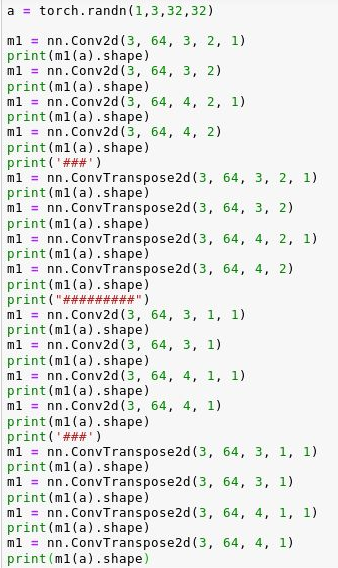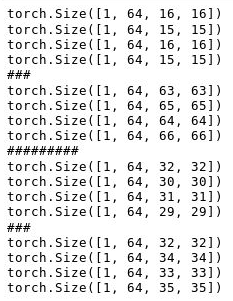12. specify GPU:

# in model
class Discriminator(nn.Module):
def __init__(self, ngpu): # ngpu: number of gpu
super(Discriminator, self).__init__()
self.ngpu = ngpu
self.main = nn.Sequential(
# input is (nc) x 64 x 64
nn.Conv2d(nc, ndf, 4, 2, 1, bias=False),
nn.LeakyReLU(0.2, inplace=True),
# ...
# state size. (ndf*8) x 4 x 4
nn.Conv2d(ndf * 8, 1, 4, 1, 0, bias=False),
nn.Sigmoid()
)
def forward(self, input):
if self.ngpu > 1:
output = nn.parallel.data_parallel(self.main, input, range(self.ngpu))
else:
output = self.main(input)
return output

13. cuda() :

device = torch.device("cuda:0" if torch.cuda.is_available() else "cpu")
model = model.to(device)
features = features.to(device)
targets = targets.to(device)


https://discuss.pytorch.org/t/how-to-check-if-model-is-on-cuda/180/7

Copies parameters and buffers from state_dict into this module and its descendants. If strict is True, then the keys of state_dict must exactly match the keys returned by this module’s state_dict() function.

netD = Discriminator(ngpu).to(device)
netD.apply(weights_init)

if opt.ResumeNetD = 'True':
print(netD)


https://pytorch.org/docs/stable/torchvision/datasets.html

15. nn.Sequential(*args) : A sequential container. Modules will be added to it in the order they are passed in the constructor. Alternatively, an ordered dict of modules can also be passed in.

# Example of using Sequential
model = nn.Sequential(
nn.Conv2d(1,20,5),
nn.ReLU(),
nn.Conv2d(20,64,5),
nn.ReLU()
)
# Example of using Sequential with OrderedDict
model = nn.Sequential(OrderedDict([
('conv1', nn.Conv2d(1,20,5)),
('relu1', nn.ReLU()),
('conv2', nn.Conv2d(20,64,5)),
('relu2', nn.ReLU())
]))


In user-defined models, use this function in __init__() :

class Generator(nn.Module):
def __init__(self, ngpu):
super(Generator, self).__init__()
self.ngpu = ngpu
self.main = nn.Sequential(
# input s Z, going into a convolution
nn.ConvTranspose2d(nz, ngf * 8, 4, 1, 0, bias=False),
nn.BatchNorm2d(ngf * 8),
nn.ReLU(True),
# state size. (ngf*8) x 4 x 4
nn.ConvTranspose2d(ngf * 8, ngf * 4, 4, 2, 1, bias=False),
nn.BatchNorm2d(ngf * 4),
nn.ReLU(True),
# state size. (ngf*4) x 8 x 8
nn.ConvTranspose2d(ngf * 4, ngf * 2, 4, 2, 1, bias=False),
nn.BatchNorm2d(ngf * 2),
nn.ReLU(True),
# state size. (ngf*2) x 16 x 16
nn.ConvTranspose2d(ngf * 2, ngf, 4, 2, 1, bias=False),
nn.BatchNorm2d(ngf),
nn.ReLU(True),
# state size. (ngf) x 32 x 32
nn.ConvTranspose2d(ngf, nc, 4, 2, 1, bias=False),
nn.Tanh()
# state size. (nc) x 64 x 64
)
def forward(self, input):
if input.is_cuda and self.ngpu > 1:
output = nn.parallel.data_parallel(self.main, input, range(self.ngpu))
else:
output = self.main(input)
return output

16. torch.stack( (tensor1, tensor2) ) : tensor of tensors

>>> a = torch.tensor([1,2])
>>> b = torch.tensor([2,3])
>>> torch.stack((a,b))
tensor([[1, 2],
[2, 3]])
>>> torch.cat((a,b))
tensor([1, 2, 2, 3])

17. tensor.backward() :

>>> x = torch.tensor((),dtype = torch.float32,requires_grad=True)
>>> y = x*2 # 1,2
>>> y.backward(retain_graph=True)
>>> y.backward(retain_graph=True)
>>> y.backward()
>>> y.backward()

tensor([2.])
tensor([6.])
RuntimeError: Trying to backward through the graph a second time, but the buffers have already been freed. Specify retain_graph=True when calling backward the first time.


see retain_graph=True below.

18. backward (retain_graph=True )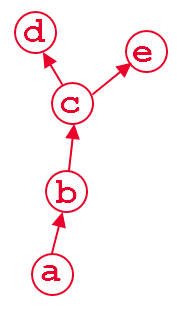need d(d)/d(a) and d(e)/d(a):

from torch.autograd import Variable
b = a**2
c = b*2
d = c.mean()
e = c.sum()
d.backward(retain_graph=True) # fine
e.backward(retain_graph=True) # fine
d.backward() # also fine
e.backward() # error will occur!


when we do d.backward(), that is fine. After this computation, the part of graph that calculate dwill be freed by default to save memory. So if we do e.backward(), the error message will pop up. In order to do e.backward(), we have to set the parameter retain_graph to True in d.backward()

a real use case is multi-task learning where you have multiple loss which maybe be at different layers. Suppose that you have 2 losses: loss1 and loss2 and they reside in different layers. In order to backprop the gradient of loss1 and loss2 w.r.t to the learnable weight of your network independently . You have to use retain_graph=True in backward() method in the first back-propagated loss:

loss1.backward(retain_graph=True)
loss2.backward() # now the graph is freed, ready for next process of batch SGD
optimizer.step() # update the network parameters


https://stackoverflow.com/questions/46774641/what-does-the-parameter-retain-graph-mean-in-the-variables-backward-method

More example:• This will work when: final_loss = loss1+loss2. And it is we need to update both model 1 and model 2 according to this final loss.
• This does not work when: we need to update model 1 according to only loss 1 and update model 2 according to loss1+loss2 (or only loss 2). This is what we said: update models separately. Then this approach will introduce loss 2 when updating model 1, which is not intended.

https://discuss.pytorch.org/t/what-exactly-does-retain-variables-true-in-loss-backward-do/3508/12

19. tensor.detach()

>>> x = torch.tensor((),dtype = torch.float32,requires_grad=True)
>>> y = x**2 # 1,2x
>>> z = 2*y # 2,2
>>> w= z**3 # 8,3z**2
>>> # detach it, so the gradient w.r.t p does not effect z!
>>> p = z.detach()
>>> q = torch.tensor((),dtype = torch.float32, requires_grad=True)
>>> pq = p*q
# Gragh:
# x --> y --> z --> w
#         --> p --> pq
>>> pq.backward(retain_graph=True)
>>> w.backward()

None
tensor([48.])

# replace p = z.detach() with:
>>> p = z
tensor([8.]) # affected
tensor([56.]) # accummulated


more summary:

1. tensor.detach() creates a tensor that shares storage with tensor that does not require grad. tensor.clone()creates a copy of tensor that imitates the original tensor’s requires_gradfield. You should use detach() when attempting to remove a tensor from a computation graph, and clone as a way to copy the tensor while still keeping the copy as a part of the computation graph it came from.
2. tensor.data returns a new tensor that shares storage with tensor. However, it always has requires_grad=False (even if the original tensor had requires_grad=True
3. You should try not to call tensor.data in 0.4.0. What are your use cases for tensor.data?
4. tensor.clone() makes a copy of tensor. variable.clone() and variable.detach()in 0.3.1 act the same as tensor.clone() and tensor.detach() in 0.4.0.

https://discuss.pytorch.org/t/clone-and-detach-in-v0-4-0/16861

## utils

1. save image patch as grad image

import torchvision.utils as vutils

fixed_noise = torch.randn(batchSize, nz, 1, 1).to(device)
# in train loop
fake = netG(fixed_noise)
vutils.save_image(fake.detach(),
'%s/find_para_dim64%d.png' % (outfile_path, epoch),
normalize=True)

2. datasets

https://pytorch.org/docs/stable/torchvision/datasets.html

4. custom dataset examples

https://github.com/utkuozbulak/pytorch-custom-dataset-examples

5. transforms.Compose([])

transform=transforms.Compose([
transforms.Resize(256),
transforms.RandomHorizontalFlip(),
transforms.ToTensor(),
transforms.Normalize((0,), (1,)),
])


https://pytorch.org/docs/0.4.0/torchvision/transforms.html

https://pytorch.org/docs/stable/torchvision/transforms.html

• torchvision.transforms.CenterCrop(size) : Crops the given PIL Image at the center.
• torchvision.transforms.FiveCrop(size) : Crop the given PIL Image into four corners and the central crop
• torchvision.transforms.RandomCrop(size) : Crop the given PIL Image at a random location.
• torchvision.transforms.Resize(size, interpolation=2) : Resize the input PIL Image to the given size. If size is a sequence like (h, w), output size will be matched to this. If size is an int, smaller edge of the image will be matched to this number. i.e, if height > width, then image will be rescaled to (size * height / width, size)

Notice:

‘transforms’ only perform on PIL images. For example when building dataset. It cannot perform on tensors:

import torch
import torchvision.transforms as transforms
b = torch.ones(1,10,10)
transforms.Resize(5,5)(b)

Traceback
TypeError: img should be PIL Image. Got <class 'torch.Tensor'>


This issue can be solved by transforming tensor to PIL beforehand:

import torch
import torchvision.transforms as transforms
import torchvision.transforms.functional as F
a = torch.ones(2,6,6)
b = F.to_pil_image(a)
c = transforms.Resize(3,3)(b)


But still not working perfectly: we can’t see the output result c directly.

Notice: ‘transforms’ are very powerful. But it performs on PIL image. That is why we normally can’t see the direct result just like other functions:But the above issue can be solved by converting it back to tensor:

c = transforms.ToTensor()(transforms.Resize(3,3)(b))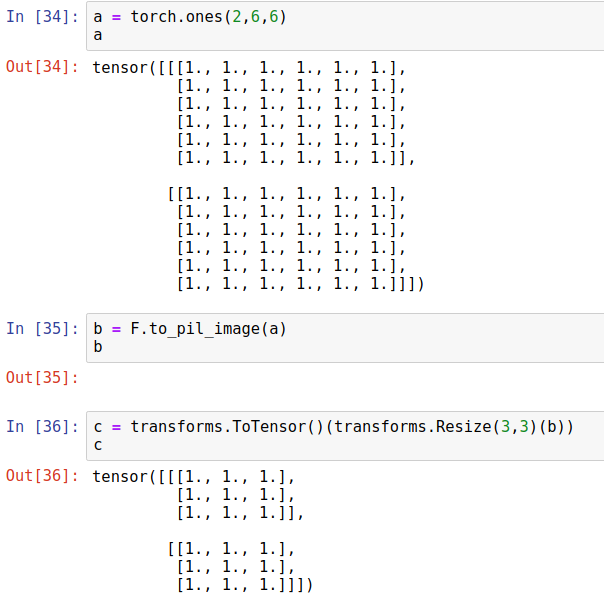Now: Enjoy the amazing power provided by ‘torchvision.transforms’ !

## Datasets

### CIFAR-10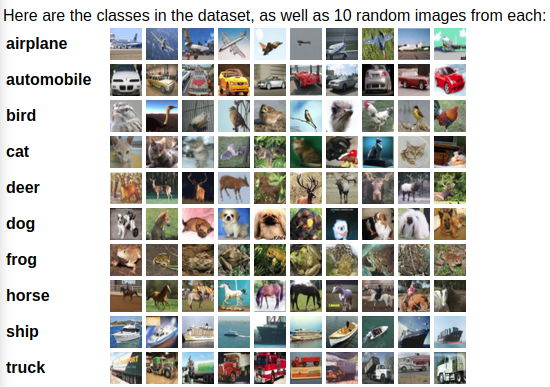def unpickle(file):
import pickle
with open(file, 'rb') as fo:
return dict


http://www.cs.utoronto.ca/~kriz/cifar.html

>>> data_dict = unpickle("data_batch_1")
>>> print(data_dict[b'batch_label'])
>>> print(data_dict[b'labels'][12:14])
>>> print(data_dict[b'data'].shape)
>>> print(data_dict[b'filenames'][12:14])
b'training batch 1 of 5'
[7, 2] # number of labels from 0 to 9: horse, bird
(10000, 3072)
[b'quarter_horse_s_000672.png', b'passerine_s_000343.png']


Some strange things:

img = np.ones((32,32,3)).astype('uint8')
img[:,:,0] = data_dict[b'data'][:1024].reshape(32,32)
img[:,:,1] = data_dict[b'data'][1024:2048].reshape(32,32)
img[:,:,2] = data_dict[b'data'][2048:3072].reshape(32,32)
plt.axis('off')
plt.imshow(img)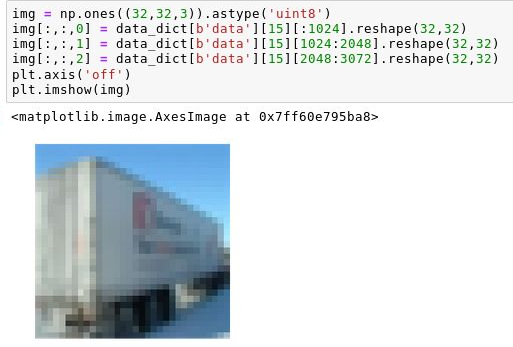img = np.ones((3,1024)).astype('uint8')
img[0,:] = data_dict[b'data'][:1024]
img[1,:] = data_dict[b'data'][1024:2048]
img[2,:] = data_dict[b'data'][2048:3072]
img = np.rot90(img,3)
img = img.reshape(32,32,3)
plt.axis('off')
plt.imshow(img)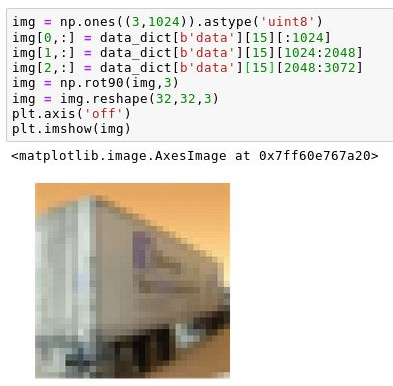import pickle
from torch.utils.data import Dataset
import os
dataroot = 'common/user/ks1418'
batchSize = 64
def get_img(img_data):
img = np.array([img_data[:1024].reshape(32,32),img_data[1024:2048].reshape(32,32),img_data[2048:3072].reshape(32,32)])
#because torch use C x H x W.
return img
def get_dataset():
dataset = []
files = ["data_batch_1","data_batch_2","data_batch_3","data_batch_4","data_batch_5"]
for file in files:
with open(os.path.join(dataroot,file), 'rb') as fo:
for i in range(len(data_dict[b'data'])):
if data_dict[b'labels'][i] == int(7): # only use 'Horse' to train
img_data = data_dict[b'data'][i]
dataset.append((torch.tensor(get_img(img_data),dtype = torch.float32),torch.tensor(8,dtype=torch.float32))) # items in dataset should by tuples of tensor: first element is image, second is label (not used but it needs to be a tuple)
return dataset
class MyDataSet(Dataset):
def __init__(self):
self.dataset = get_dataset() # read in all training images
def __getitem__(self, index):
return self.dataset[index]  # return by index
def __len__(self):
return len(self.dataset)

dataset = MyDataSet()
dataset=dataset,      # torch TensorDataset format
batch_size=batchSize,      # mini batch size
shuffle=True,
num_workers=2,
)


## trouble shooting

1. Input type (torch.cuda.DoubleTensor) and weight type (torch.cuda.FloatTensor) should be the same

Try moving the data to torch.float, i.e data.to(device=device, dtype=torch.float).

The size of tensor and size of CNN are not proper. take care of the shape of tensor. Usually, tensor is too small.

>>> m = torch.nn.Conv2d(3, 5, 4, 1).to(device)
>>> good = torch.randn((1, 3, 10, 10)).to(device)
>>> small = torch.randn((1, 3, 2 ,2)).to(device)
>>> print(m(good).shape)
>>> m(small)

torch.Size([1, 5, 7, 7])

• When stride = 1 to keep same image size, only use kernel size = 3
• when stride =2 and do conv or convtranspose to scale up/down: conv : free, convtranspose: only kernel 4
• kernel size = 4 is safe in both conv and convtranspose
• conv:
• use padding = 1 to get input/2
• use padding = 0 to get input/2-1
• convtranspose:
• use padding = 1 to get 2*input
• use padding =0 to get 2*input+2)
• kernel size = 3 is only applicable in conv, use padding = 1

If you see Loss is always 0, and results are almost white noise, it probably because of gradient vanish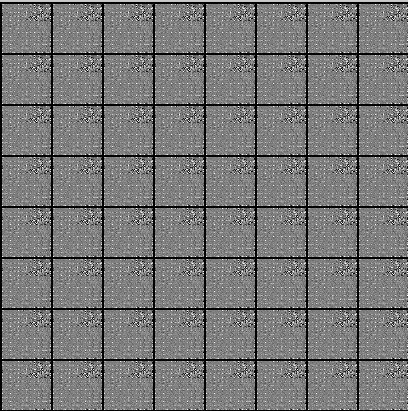Use spectral norm after each convolution to control the size of gradient update

from torch.nn.utils import spectral_norm

# ... in sequential
# ...


But this leads to slower training. At the beginning, it seems to have collapsed, but gradually different results come out finally. Also, deeper CNN trains much slower than shallow CNN, and are more likely to be considered as collapse by mistake. (Actually, it is indeed more likely to collapse, but at least it won’t suffer from gradient vanish) (left is one layer deeper than right, both of them uses spectral norm.)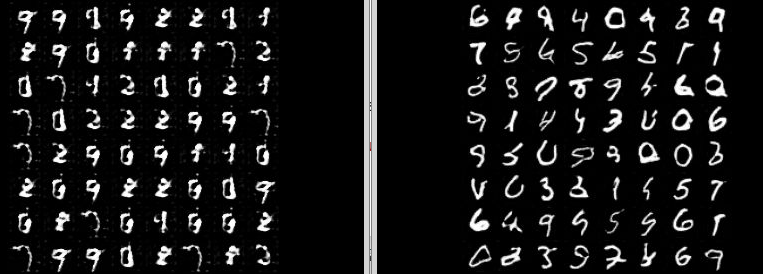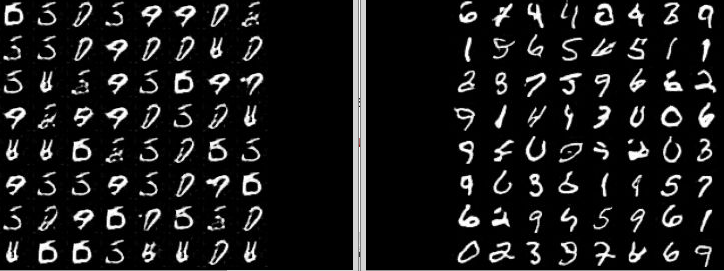4. model collapse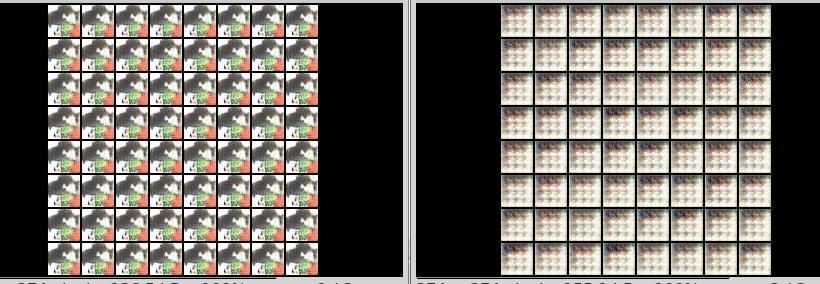Deeper and more complex model tends to collapse.

WGAN solved this issue almost completely.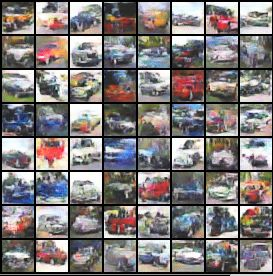Train D more:

for epoch in range(niter):
# inside each batch
img = 0
for j in range(5): # train D 5 times more than G
img = img+1
if img >1:
break
# example output of above inner loop
tensor()
tensor()
tensor()
tensor()

5. About batch size : avoid to big batch size when the training data set is small.

Eg: 360 coast images training data set, different batch size at the same training time:

• batch size 64

•• batch size 16

•6. one of the variables needed for gradient computation has been modified by an inplace operation

This means some variable A is rewritten, then in backward() , its original value is needed.

output = netD(real_img)
errD_real = criterion(output, label)

noise = torch.randn(batch_size, nz, 1, 1).to(device)#,dtype=torch.float32)
fake = netG(noise)
output = netD(fake.detach()) #rewrite
errD_fake = criterion(output, label)
errD = errD_real+errD_fake
errD.backward() # need original value


To make life easier, call .backward() after calculation each item of loss function.

errD_real = criterion(output, label)
errD_real.backward()
# ...
errD_fake = criterion(output, label)
errD_fake.backward()
optimizerD.step()

7. ValueError: only one element tensors can be converted to Python scalars:

Notice: connot convert list of tensors to tensor of tensors

>>> a = torch.tensor([1,2,3])
>>> b = torch.tensor([2,4,3])
>>> c = [a,b]
>>> torch.tensor(c)
ValueError: only one element tensors can be converted to Python scalars
>>> c = torch.zeros(2,3)
>>> c = a
>>> c = b
>>> c
tensor([[1., 2., 3.],
[2., 4., 3.]])

8. torchvision normalize does not working:

https://stackoverflow.com/questions/53332663/torchvision-0-2-1-transforms-normalize-does-not-work-as-expected/53337082#53337082

The transforms will not be called until the dataloader derived form the dataset (transform involved) is iterated.

9. torchvision.datasets.ImageFolder:

RuntimeError: Found 0 files in subfolders of: /common/users/ks1418/images/coast. Supported extensions are: .jpg,.jpeg,.png,.ppm,.bmp,.pgm,.tif

The image path is correct but still cannot find the training images. The key is the folder structure: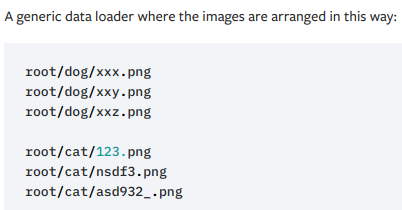At least one subfolder is needer. Change imaga_path to ‘ /common/users/ks1418/images/ ‘ will work.

10. CUDA out of memory, specify GPU

>>> CUDA_VISIBLE_DEVICES=0,2 python landscape_no_seg.py
# use cuda parallel in code and sed ngpu=2
RuntimeError: CUDA out of memory. Tried to allocate 2.00 GiB (GPU 1; 10.91 GiB total capacity; 8.79 GiB already allocated; 1.59 GiB free; 2.43 MiB cached) (malloc at /opt/conda/conda-bld/pytorch_1550780889552/work/aten/src/THC/THCCachingAllocator.cpp:231)

>>> torch.cuda.device_count()
4
# It is said in the Rutgers CS official doc that we have 8 GPU per linux machine..
# But one student can only use 4 of them. So cuda device_count() is 4.


CUDA will enumerate the visible devices starting at zero. In the last case, devices 0, 2, 3 will appear as devices 0, 1, 2.

So if you do CUDA_VISIBLE_DEVICES=2, then your gpu #2 will be denoted as gpu:0 inside tensorflow.

The 2 GB in  Tried to allocate 2.00 GiB is related to model size, not batch size.

I reduced the number of filters and the above problem solved.

More:

• Smaller batch size

• torch.cuda.empty_cache() every few minibatches

• CUDA_VISIBLE_DEVICES=0 python find_para_landscape.py

multi GPU:

1. CUDA_VISIBLE_DEVICES=2,3 python myscript.py # GPU 2 is cuda 0, GPU 3 is cuda 1 2. net = torch.nn.DataParallel(model, device_ids=[0, 1])
11. NVIDIA memory not deallocated after keyboard interrupt

Check the GPU state: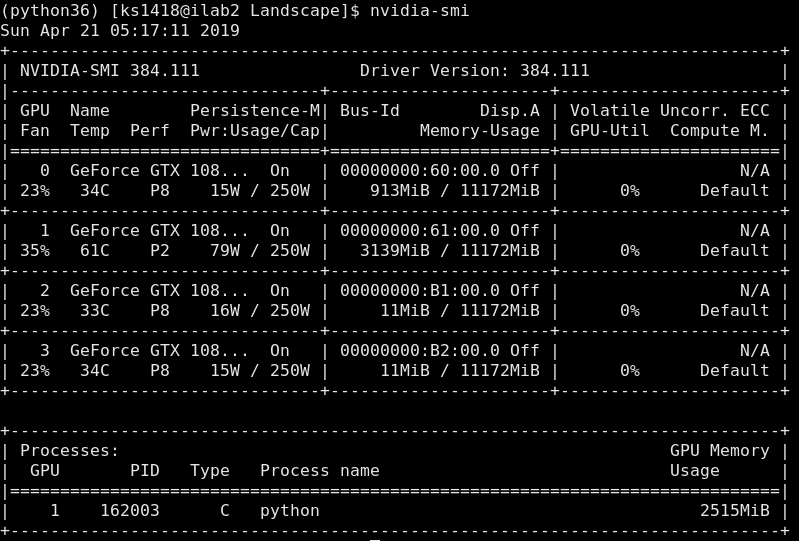Chose GPU with more memory: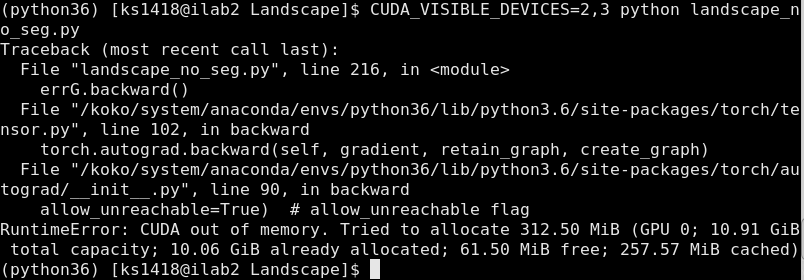Check Linux process: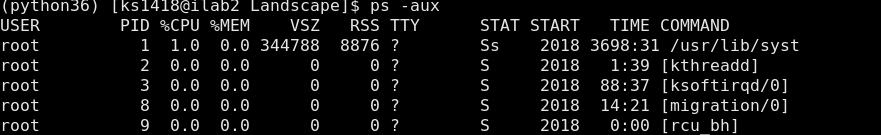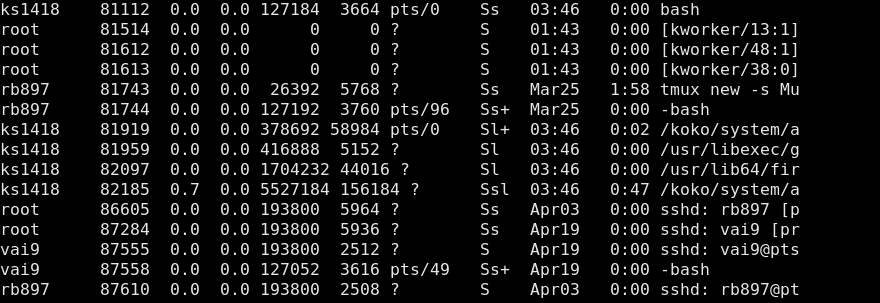• RSS resident set size, the non-swapped physical memory that a task has used (in kiloBytes).
• VSZ virtual memory size of the process in KiB (1024-byte units).

Kill process using Linux command: https://blog.csdn.net/andy572633/article/details/7211546

• ps -aux same as ps -ef : see all processes

•  ps -aux grep ks1418 : see processes only contain ‘ks1418’. ( ‘ ’ means Pipeline, the result of left is transfer to grep to filter keywords)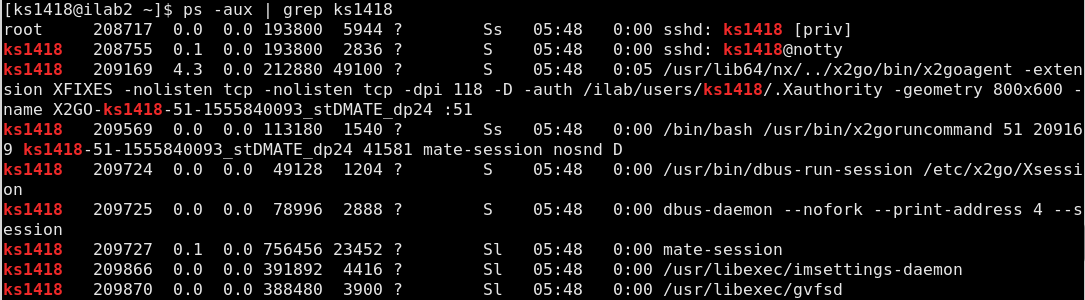When investigating, we found that there’s actually a bug in python multiprocessing that might keep the child process hanging around, as zombie processes. It is not even visible to nvidia-smi.

The solution is kill all python, or to ps -elf | grep python and find them and kill -9 [pid] to them.

https://discuss.pytorch.org/t/pytorch-doesnt-free-gpus-memory-of-it-gets-aborted-due-to-out-of-memory-error/13775/12

try:

• Log out iLab will kill all ks1418 related processes and release some of GPU memory requested by my keyboard-interrupted python programs.

• Change model structure and adopt a smaller model.

• Try to specify GPU id by:

CUDA_VISIBLE_DEVICES=2 python myscript.py # if no multi GPU in code

12. transforms centercrop and resize:

Training images are not of same size. Some 2048 X 2048, some 356 X 256. Some of them may not be square but rectangular. We would like to use 64 x 64 pixel images to test our idea. How to deal with this training image set?

imagenet_data = dataset.ImageFolder(dataroot,transform=transforms.Compose([
transforms.CenterCrop(64),
transforms.Resize(64)
transforms.ToTensor(),
transforms.Normalize((0,), (1,)),
]))
batch_size=batchSize,
shuffle=True,
num_workers=nworkers)
# This will NOT work!
# I waited for a long time with this code, but only got a lot of bad images.

# The key point is "centercrop and resize"
# Remember centercrop will only cut a small part from the center of our image. It is NOT maximum center cut and resize!
# SO if we call 'centercrop' before resize, we will get a lot of non-sence training images.

# The following is the correct version:
imagenet_data = dataset.ImageFolder(dataroot,transform=transforms.Compose([
transforms.Resize(64), # resize first
transforms.CenterCrop(64), # centercrop second.
transforms.ToTensor(),
transforms.Normalize((0,), (1,)),
]))



Also, this code is not perfect, because it will use these ‘transforms compose’ every time it train with one image.

I found it much faster if we prepare 64 x 64 training images beforehand:

# use torchvisio transforms to prepare training images
import os
import torch.utils.data as Data
import torchvision.datasets as dataset
import torchvision.transforms as transforms
import torchvision.utils as vutils

dataroot = '/common/users/ks1418/images/no_seg/street' # original images are in it subfolder 'image'. Attention: we need at least one subfolder when using ImageFolder.
outf = '/common/users/ks1418/images/no_seg/street_64/image' # save new images
names = []
for img in os.listdir(os.path.join(dataroot,'image')):
names.append(img) # preserve the image names. Attention: this code will not keep image name unchanged. The permutation of new image names are different from before.
imagenet_data = dataset.ImageFolder(dataroot,transform=transforms.Compose([
transforms.Resize(64),
transforms.CenterCrop(64),
transforms.ToTensor(), #This IS Needed !!! Otherwise, you got "tensor or list of tensors expected, got <class 'PIL.Image.Image'>" when calling "vutils.save_image".v
]))
for cnt in range(len(imagenet_data)):
img = imagenet_data[cnt]
vutils.save_image(img,os.path.join(outf,image_names[cnt]),normalize=False)

# Or: for paired images: in 'dataroot', we have two folders: image, annot
for cnt in range(int(len(imagenet_data)/2)):
img = imagenet_data[int(len(imagenet_data)/2)+cnt]
vutils.save_image(img,os.path.join(outf,'image',image_names[cnt]),normalize=False)
img = imagenet_data[cnt]
vutils.save_image(img,os.path.join(outf,'annot',image_names[cnt]),normalize=False)
# imagenet_data contains both image and annot. First half are annots, whose form is (annot_image_tensor,tensor). The last half are images, whose form is (image_tensor,tensor)


Notice: You may not use ‘transforms’ in CNN layers. Because:

• You have to convert tensor to PIL before calling ‘transforms’

• You would need to copy tensor to CPU for the above process. This is computationally very expensive.

• Use nn.Upsample instead. (It is also capable for down sampling).

nn.Upsample(size=16, mode=’bilinear’)( tensor1 )

13. Multi GPU not faster.

netG = Generator()
netD = Discriminator()

if torch.cuda.device_count() > 1:
print("Let's use", torch.cuda.device_count(), "GPUs!")
netD = nn.DataParallel(netD)
netG = nn.DataParallel(netG)

netG.to(device)
netG.apply(weights_init)
netD.to(device)
netD.apply(weights_init)


Attention:

If you use batch_size=30 using a single GPU, then when you use DataParallel with 3 GPUs, you should use batch_size=90 to make a fair comparison. The point of using DataParallel is that you can use a larger batch_size which then requires less number of iterations to complete one full epoch.

## …

Questions:

1. torch.nn.Conv2d`(in_channels, out_channels, kernel_size, stride=1, padding=0, dilation=1, groups=1, bias=True)

groups (int, optional) – Number of blocked connections from input channels to output channels. Default: 1

3. input image normalization. 0 mean 1 var works perfectly and the result image is not bluish. Why they use 0.5,0.5?

4. torch.nn.functional.interpolate and upsample

Main References:

https://pytorch.org https://github.com https://discuss.pytorch.org https://stackoverflow.com https://blog.csdn.net